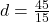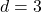## Sample A has a mass of 40g and a volume of 10cm^3. Sample B has a volume of 15cm^3 and a mass of 45g. Which sample has a greater density? Sh

Question

Sample A has a mass of 40g and a volume of 10cm^3. Sample B has a volume of 15cm^3 and a mass of 45g. Which sample has a greater density? Show your work.​

in progress 0
3 weeks 2021-08-23T23:35:51+00:00 1 Answers 0 views 0

1. The Answer Is Sample A

Density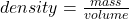Input The Formula For Both Equations To Find Density.

Sample A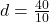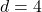Sample B –